Actions

Introduction

Actions provide a way to react to events in the calculator, like clicks or clock ticks. An action is composed of one or more update rules that each specify a new value for a variable, depending on a specific event within the expression list or the graph paper.

Use the resources below to enable Actions on your account and learn about all of the features that are available once they're enabled.

Enabling Actions

Actions are an advanced feature in our graphing calculator that you must enable at the account level.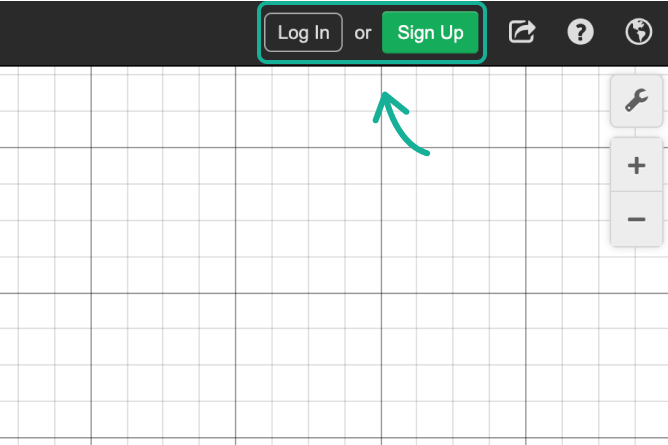Click on your name in the top right to bring up account options and click Account Settings.Go to the Advanced tab and check the Actions checkbox.

Getting Started With ActionsUse a right arrow to author an action. You can write the → symbol by typing the subtraction symbol (-) followed by a greater than sign (>).When you write an action in the expression list, make sure you enable the slider for the included variable.

The expression with the action will then display an action icon that will run the action every time you click on it.Some examples of basic actions:

Set $$a$$ to 1: $$a$$ → 1

Increment $$a$$: $$a$$ → $$a$$ + 1

Swap the values of $$a$$ and $$b$$: $$a$$ → $$b$$, $$b$$ → $$a$$

Clickable Objects

When actions are enabled, you can attach an action to graphed objects like points, curves, and images. The action will run when the object is clicked in the graphing calculator window.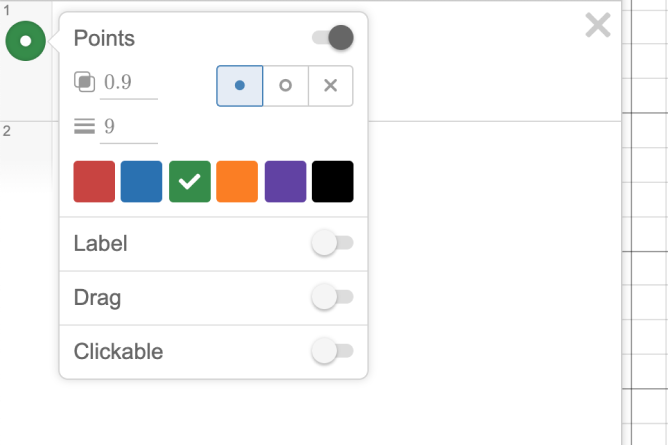Long press the expression icon to bring up the style menu.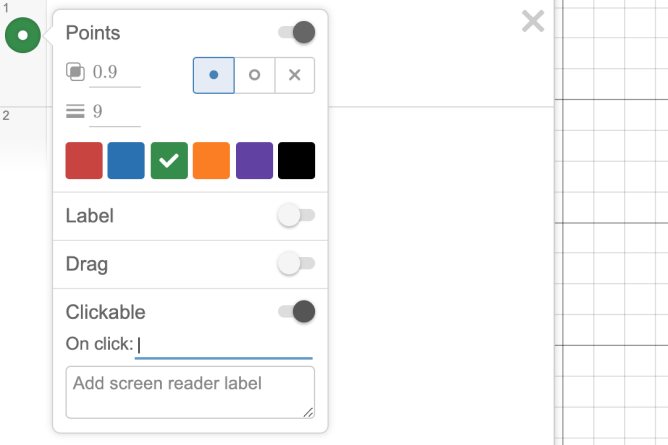Toggle Clickable to the “on” position.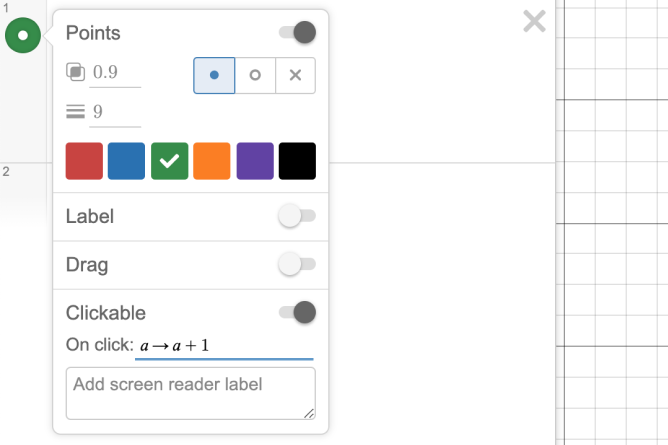In the field titled On Click, you can author an action that will be performed when the object is clicked within the graphing calculator itself.

Clickable Objects: Example One

In the example to the right, the compound inequality $$0$$ ≤ $$y$$ ≤ $$k-x^{2}$$ has been made clickable, with the action incrementing the variable $$k$$ by one with each click anywhere in the inequality.

Explore this graph at this link.Clickable Objects: Example Two

In this example, functions are defined to produce actions, and polygons are used to increment or decrement the value of a variable, which represents the slope of a graphed line.

Explore this unique implementation of actions and clickable objects at this link.The Ticker

When actions are enabled, you can specify an action that will run repeatedly at a specified interval.To add a ticker, click the Add Expression button in the top left of the expression panel of the graphing calculator. You can also type ticker into an expression line.Specify an action to run.

Optionally, specify how much time (in ms) should pass between ticks.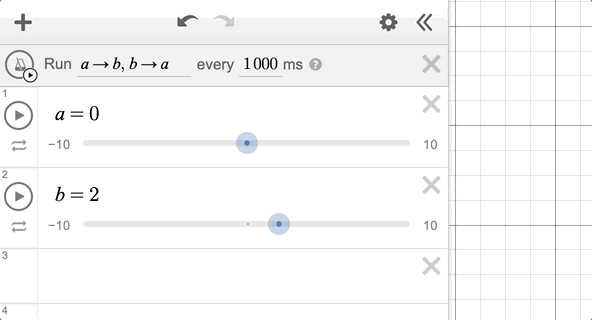Click the icon next to the ticker to run the action repeatedly over the time interval.

Actions using Variables and Function Notation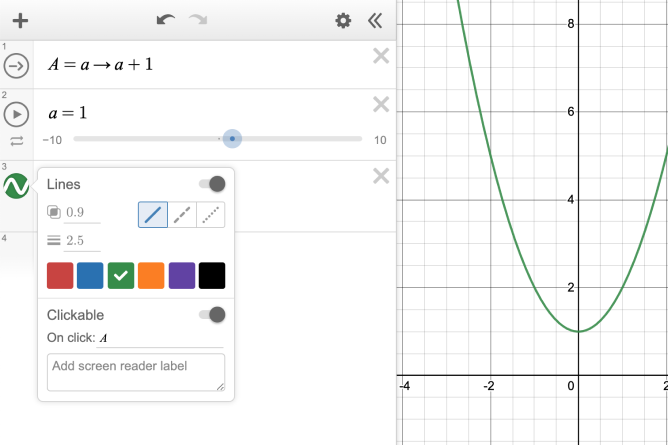Actions can be assigned to variables:

$$A = a$$ → 1

This allows attaching the same action to several objects.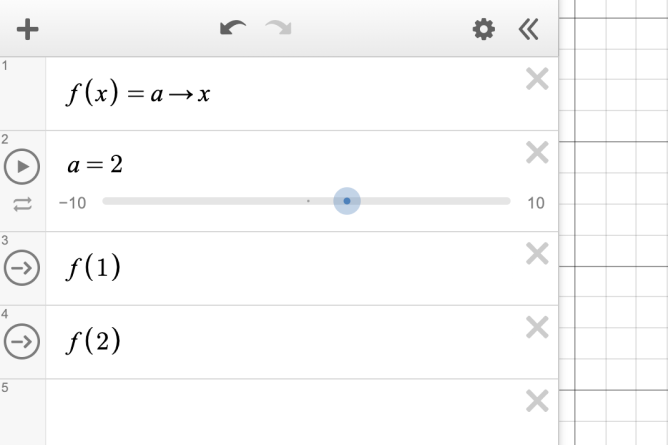Functions can also produce actions:

$$f(x) = a$$ → $$x$$

This allows making related actions, so with this definition, $$f(1)$$ and $$f(2)$$ will be two different actions that set $$a$$ to different values when they run.Piecewise expressions (using restriction notation in curly braces) allow selecting between different actions depending on a condition.

You can do things like decrement $$a$$, but only if it is positive. Or, if $$a$$ is not positive, set $$b$$ to 1, otherwise, set $$c$$ to 1.

Pro TipsWhen a click action is attached to a list, the special variable “index” represents which element of the list was clicked.In tick actions, the special variable $$dt$$ represents how much time has passed since the last tick. You can use $$dt$$ to track how long the ticker has been playing.

Click here to see an example graph that uses $$dt$$ to measure the ticker time.When random( ) is used in an action definition, a new random number will be used every time the action runs.